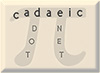True or False? Some Integer Conjectures Mike Keith

There's something satisfying about exploring some corner of the mathematical universe and discovering new facts and theorems. Even more interesting is to dig far enough to come across possible theorems whose proof is elusive. Here are a few conjectures from my ramblings among the positive integers.

### The Weighty Beast conjecture

This one relates to the number 666. First, a few definitions are required. A polygonal number is a positive integer of the form

P(k,n) = n((k - 2)n + 4 - k)/2

where k is the 'order' of the polygonal number (k=3 gives the triangular numbers, k=4 the squares, k=5 the pentagonal numbers, etc.), and n is its index. In other words, P(k,n) is the nth kth-order polygonal number. A repdigit number is an integer whose base-10 representation consists of a repeated non-zero digit (e.g., 11 or 222 or 555555). Define the weight of a polygonal number as n/k. Finally, define a repdigit polygonal number as a polygonal number that also happens to be a repdigit.

Observe that P(3,36) = 666, so 666 is a repdigit polygonal number, with weight = 36/3 = 12. Now the conjecture:

Conjecture: Among all positive integers, 666 is the repdigit polygonal number with largest weight.

This is known to be true up to 10^15.

In fact, there's an even stronger conjecture, based on looking at all the known repdigit polygonal numbers and sorting them by weight:

Conjecture: Among all positive integers, the three repdigit polygonal numbers with largest weight are:

P(3,36) = 666 (weight = 12)
P(9,44) = 6666 (weight = 4.88)
P(3,11) = 66 (weight = 3.66)

Notice all the 6's in this conjecture! This is rather weird and wonderful, since among all the repdigit polygonal numbers the frequency of occurrence of the digit 6 is not significantly greater than any other digit.

### The number 121

The number 121 has the following remarkable property: it is a perfect square, and it is a palindrome simultaneously in four of the bases in the range 2 to 10 (it's 121 in base 10, 171 in base 8, 232 in base 7, and 11111 in base 3). This leads to:

Conjecture: 121 is the only positive integer that is a perfect square and is a palindrome in four of the bases 2 through 10.

This is known to be true up to 10^13. In fact, there are only three integers (squares or not) less than 10^13 that are palindromes in four of the bases 2 to 10: 121, 373, and 786435. Are there an infinite number of integers of this type? I would conjecture "yes", but, as far as I know, this has not been proven.

### The Root/Factorial Conjecture

Here is a really wacky conjecture inspired by having spent too much time working on problems of the sort "take four 4's and some arithmetic signs and make an expression that equals X". Some work on generalizations of this problem led to a series of conjectures, the most outlandish being:

Conjecture: Any positive integer can be expressed in the form

[ sqrt(sqrt(sqrt(...sqrt(3!!!...!)))...) ]

where [ ] is the greatest-integer (floor) function. At first glance this sounds a bit preposterous, but on further thought seems probably true!  I have no idea how one might go about proving it, which as far as I know has not been done.  Is this a new conjecture, or has someone out there seen it before? Of course there's nothing special about "3" - probably any starting number will do.

Here are the only integers between 1 and 10000 that we know how to make this way:

1 = [sqrt(3)]
2 = [sqrt(3!)]
3 = 3 (and also [sqrt_5813_times(3!!!!)]
4 = ??
5 = [sqrt(sqrt(3!!))]
6 = 3!
7 = [sqrt_11_times(3!!!)]
8 = [sqrt_(K+2)_times(3!!!!!))]
15 = [sqrt_5812_times(3!!!!)]
26 = [sqrt(3!!)]
50 = [sqrt_10_times(3!!!)]
78 = [sqrt_(K+1)_times(3!!!!!)]
226 = [sqrt_5811_times(3!!!!)]
720 = 3!!
2576 = [sqrt_9_times(3!!!)]
6165 = [sqrt_K_times(3!!!!!)]

where K = 15087....12360 is an integer with 1751 digits

I computed the ones up to 3!!! by brute force, which isn't hard since 3!!! has "only" 1746 decimal digits. The ones based on 3!!!! and 3!!!!! were computed in 2002 and 2003, respectively, by Dr. Michael Hartley using Stirling approximations.  (Basically, you use Stirling to compute the intermediate results with enough precision that you can be sure of the integer part of the final expression.)  Notice that with 3!!!!! we've reached the point where not only is the factorial ridiculously large, but so is the number which says how many times to take the square root!

It's interesting that we still don't know how to make the number 4, though the chance of achieving it, by luck, with one of the next few factorials (3!!!!!! or 3!!!!!!!) is quite high - if we can figure out how to compute them.

Assuming the truth of this conjecture, Michael Hartley points out that a stronger conjecture is probably true as well: every positive integer can be expressed in this form in an infinite number of ways.